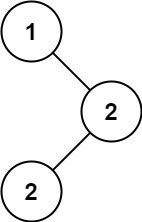# Leetcode 501 - Find mode in BST

Note:

• To avoid iterating the tree twice (1st is building it, 2nd is find the most frequent occurence), we should find the result while traversing! - Use inorder becasue it will return a sorted array.
• We set up count, maxCount, current to store states.
• When node.val === current:
• count > maxCount, then clear the result array and push the new max. Also remember to update current
• count === maxCount, push node.val into result.
• When node.val !== current:
• node is the first node (root), just push it into result.
• All element in result only appear once, so just the current one.

Given the root of a binary search tree (BST) with duplicates, return all the mode(s) (i.e., the most frequently occurred element) in it.

If the tree has more than one mode, return them in any order.

Assume a BST is defined as follows:

• The left subtree of a node contains only nodes with keys less than or equal to the node’s key.
• The right subtree of a node contains only nodes with keys greater than or equal to the node’s key.
• Both the left and right subtrees must also be binary search trees.

Example